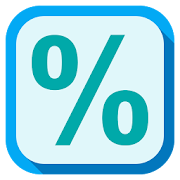Quick Percentage CalculatorEveryone
784
Calculate percentages like a pro with Quick Percentage, Now with Basic Calculator

Quick Percentage allows you to :

0. Do basic calculator operations.

You can directly input calculations like 23+23 , 15*30 , 40-20 , 10/5 and find results.

1. Calculate simple percentage.

You can calculate simple percentage.
For example, you can calculate what is the percentage of 545 out of 625.

2. Increase by percentage.

You can increase values by percentage. This tool can be used to calculate GST, VAT.
For example, you can increase 1299 by 12.5%.
Example, After adding 15% GST Product of 3000 becomes 3450.

3. Decrease by percentage / discount calculator.

You can decrease numbers by specific percentage. You can also calculate discounted price of products.
For example, you can decrease 15% from 1200. You can find the price of a product after 25% discount.

4. Find percentage value.

You can simply find percentage value of a number.
For example, you can find what is 25% of 18999.

5. Percentage change.

You can find percentage change.
For example, You can find percentage change from 1800 to 2400.

6. Tip and Split calculator.

You can calculate tip and per head contribution.
For example, You can divide the bill of 1000 in 8 people.

7. Convert fraction to percentage.

You can directly convert fractions into percentage.
For example, You can find percentage value of fraction 1/8.

8. Margin calculator.

With Margin calculator, you can find required Revenue or Selling price for achieving given Margin (%).

9. GST ( Goods and Services Tax ) Calculator

The GST Calculator allows you to,
- Add or Subtract / reduce GST Amount from Total Amount.
- View GST Tax Rates.
- View GST Amount Details.
- You can use existing GST rates or enter Custom GST Tax Rates.
- Share the GST Calculations directly from the app using mediums such as E-Mail, Text Message, Whatsapp, Facebook, Instagram or any other social media.

10. Calculate compound interest.

You can calculate compound interest.

11. Price comparison.

You can compare prices of a smaller quantity product and a bigger quantity product.

12. BMI Calculator

Calculate Body Mass Index ( BMI ) and find Ideal Weight using Broca Index.

Uses of The Quick Percentage Calculator :

1. Calculate percentages.
2. Find percentages.
3. Calculate percentage of Marks.
4. Calculate quantities.
5. Calculate discounts.
6. Calculate compound interest.
7. Convert fractions to percentages.
8. Find Revenue and Profit by using Margin.
9. You can calculate tip and per head total using Tip and Split Calculator.

The Quick Percentage Calculator offers you exclusive features like :

1. Standard Memory function keys

It has M+, M-, MC and MR buttons like a standard calculator.

2. Direct Result Sharing

You can directly share results you have calculated using a dedicated share button.

3. Direct Copy

You can directly copy results you have calculated using a dedicated copy button.

4. Directly Launch the App in any Function

You can create shortcut widgets by using which you can launch the app directly in any function you want to.

5. Basic Calculator

You can directly input calculations like 23+23 , 15*30 , 40-20 , 10/5 and find results.

Don't Forget To Like The Quick Percentage Calculator On Facebook

Try our new app - Calculator E Plus

Do let us know what do you think about the app. You can review our Percentage Calculator on Google Play or you can directly email us!
Your review will halp us to make the Calculator even better and useful.

Thanks!
Collapse

Review Policy
4.7
784 total
5
4
3
2
1

What's New

v3.1.1
★ Bug Fixes
★ Duration of Compound Interest can be set in Years, Months and Days
Collapse

Updated
February 4, 2019
Size
2.2M
Installs
100,000+
Current Version
3.1.1
Requires Android
4.0 and up
Content Rating
Everyone
Permissions
Offered By